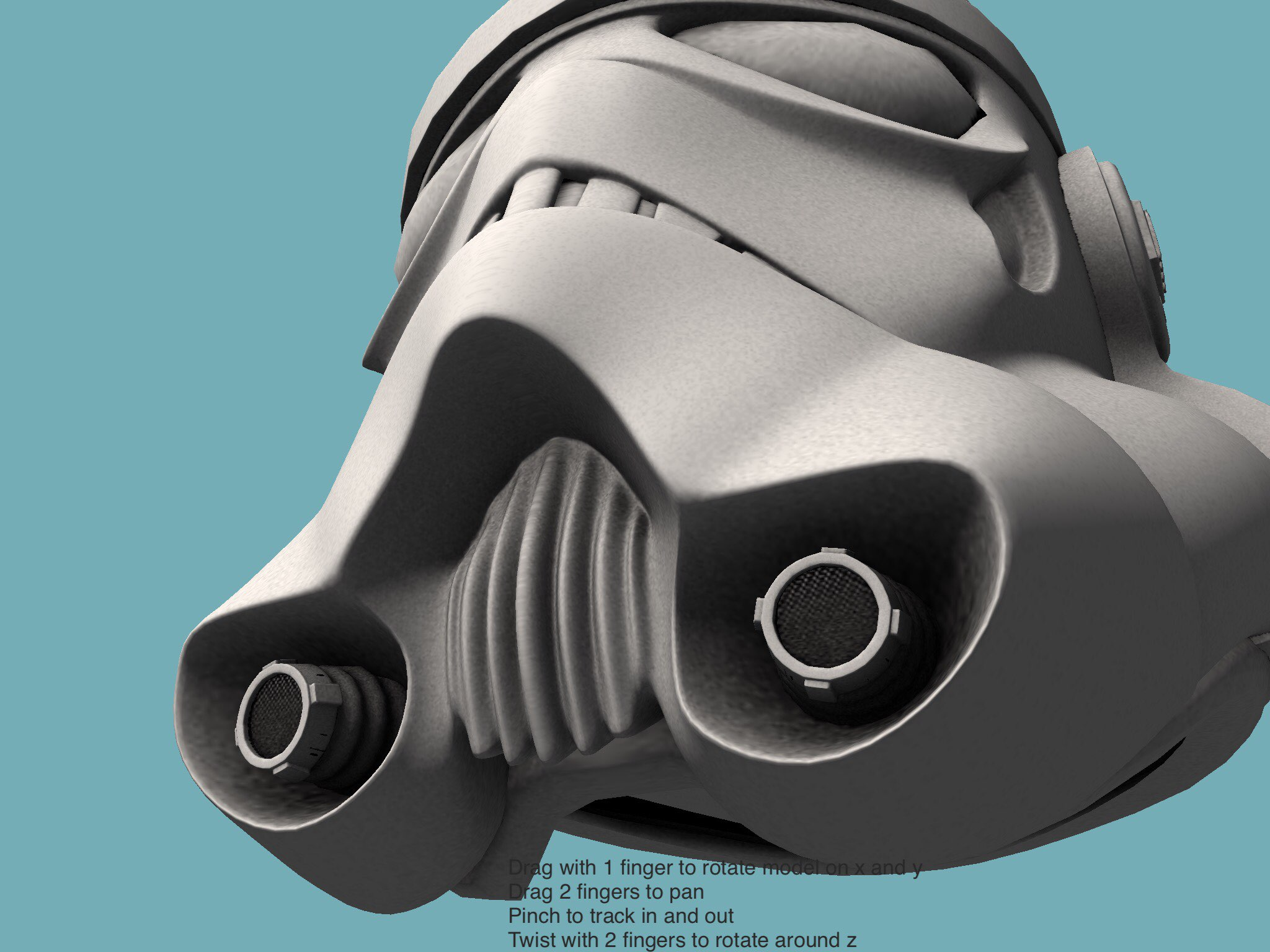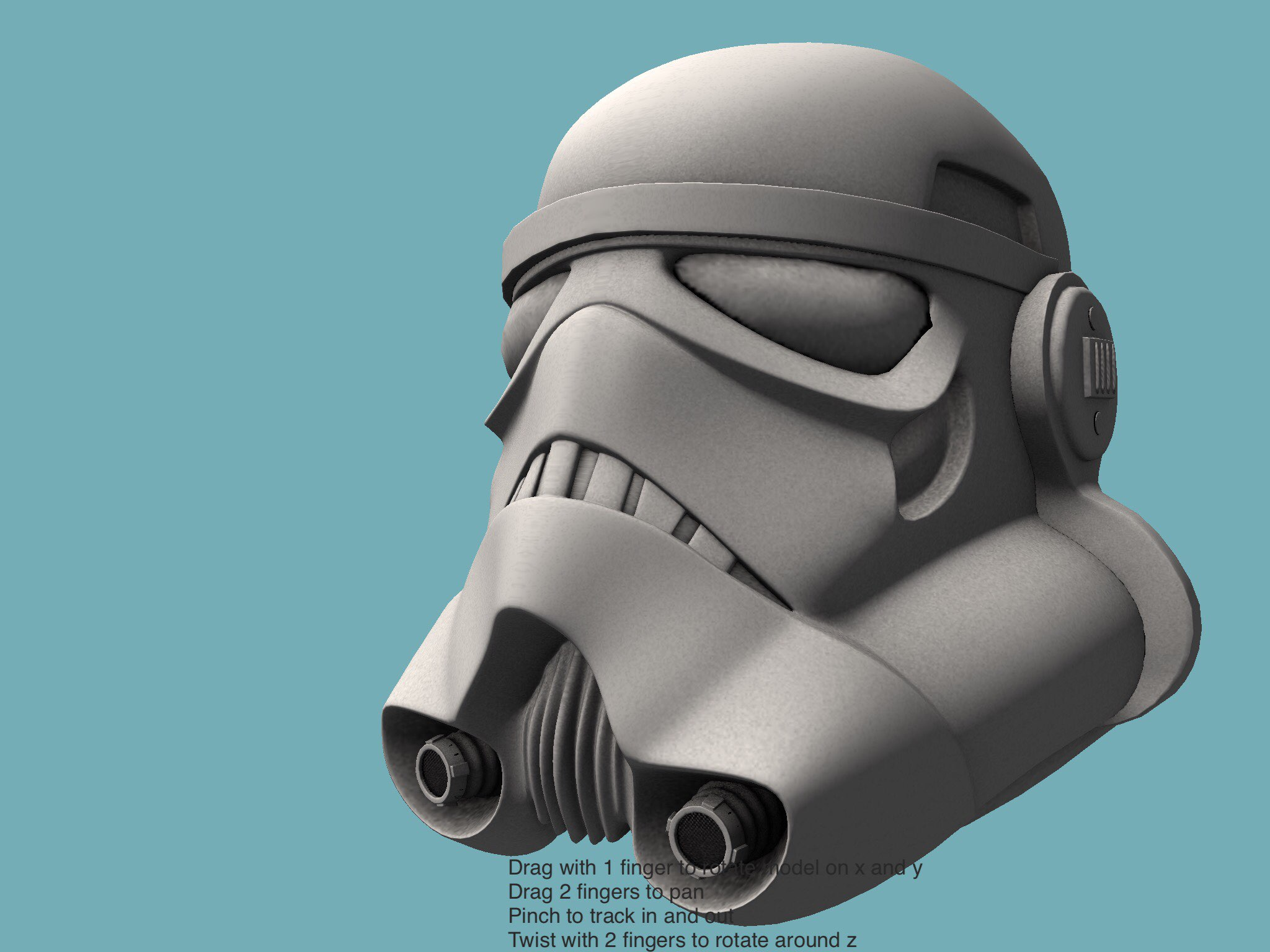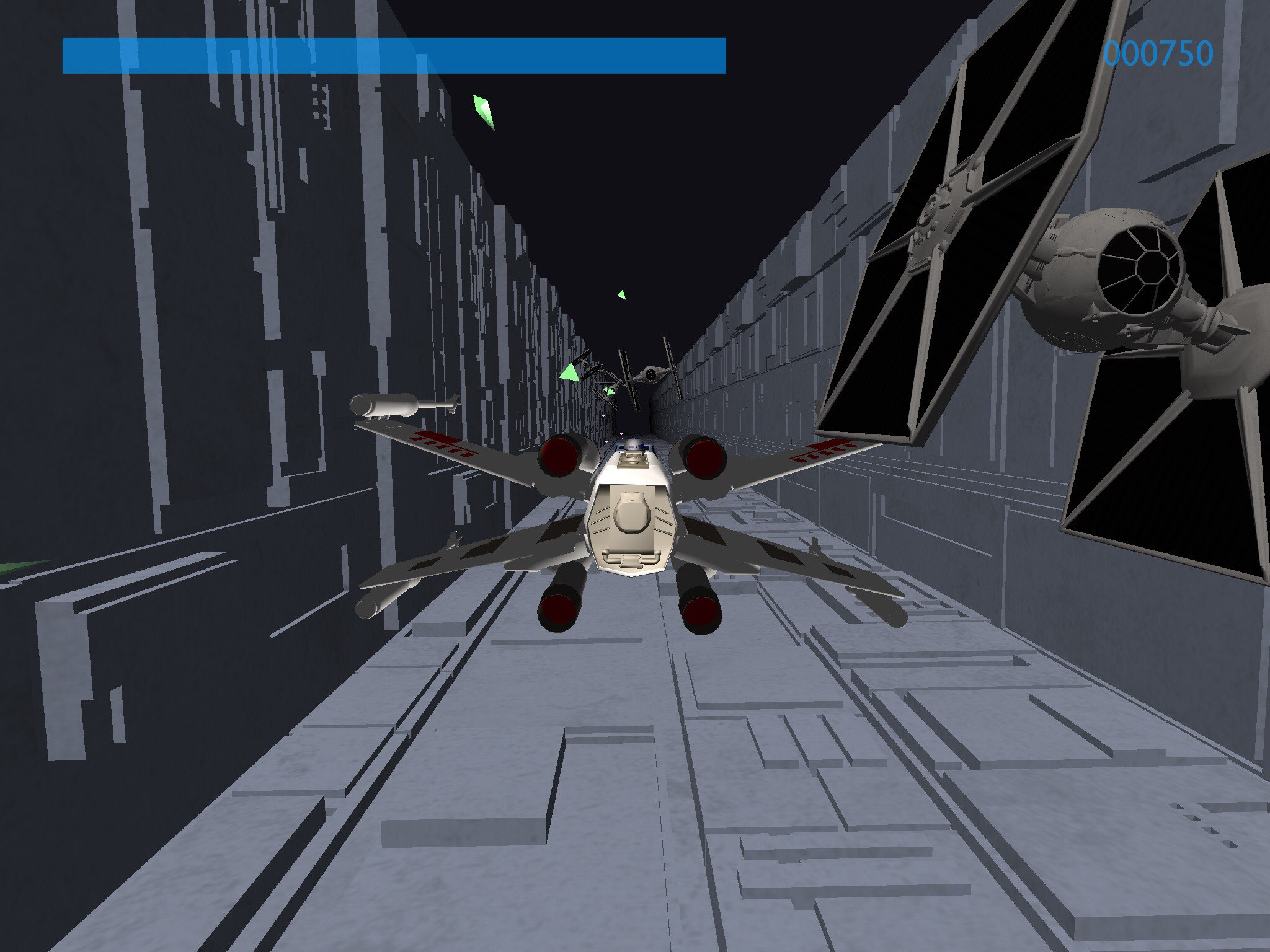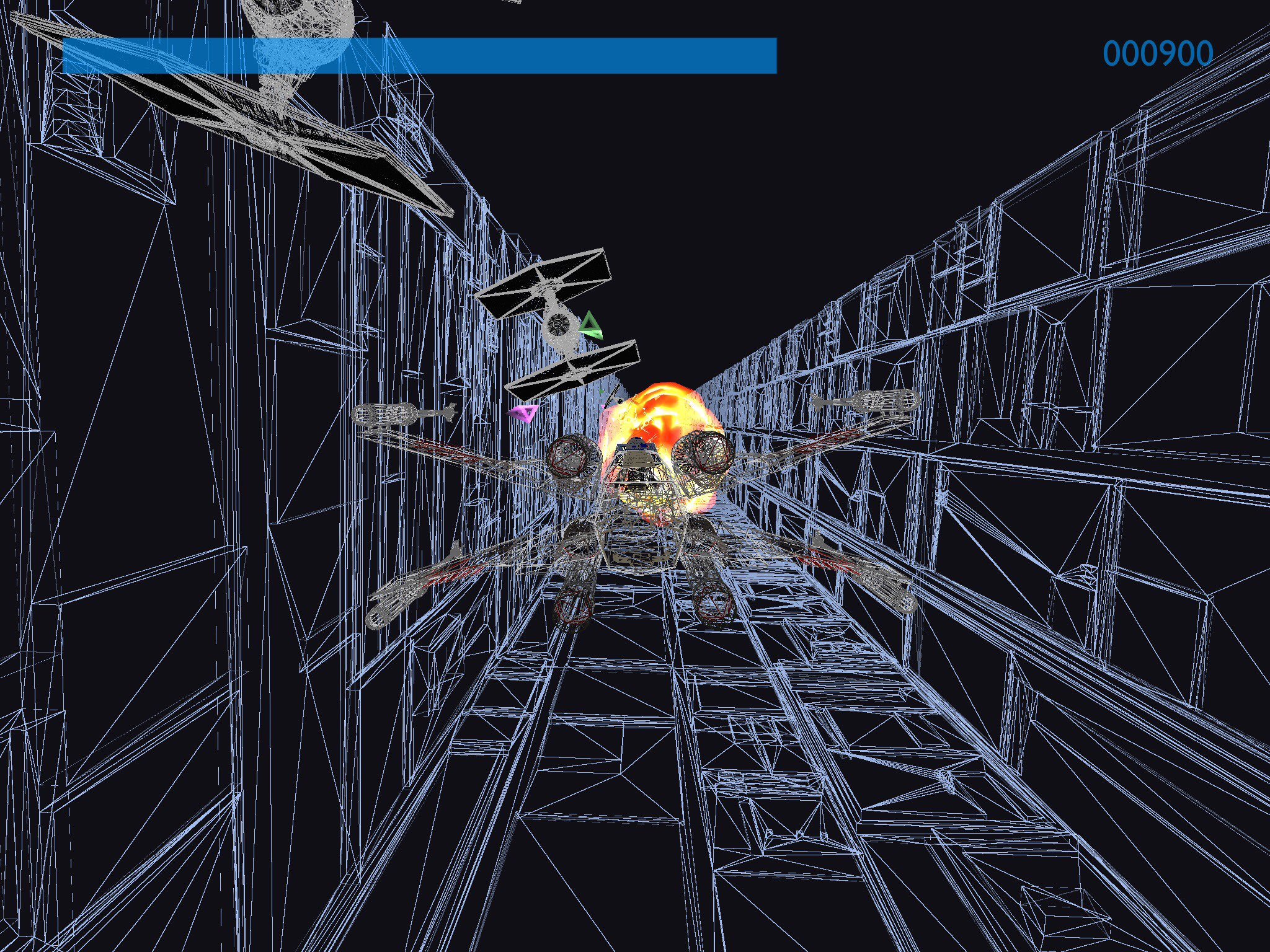#### Howdy, Stranger!

It looks like you're new here. If you want to get involved, click one of these buttons!

# Another competition, a long time ago, in a galaxy far away...

## Comments

• Posts: 871

Turning bb8 into a game - tap to jump. Something up with the physics though - sometimes falls through the platforms, sometimes passes through the screen edge. What am I missing?

``````-- Bb8
--By West
displayMode(FULLSCREEN)
-- Use this function to perform your initial setup
function setup()
size=25
bbody=physics.body(CIRCLE,size)
bbody.x=WIDTH*0.05
bbody.y=HEIGHT
bbody.bullet=true

physics.body(EDGE,vec2(0,0),vec2(0,HEIGHT))
physics.body(EDGE,vec2(WIDTH,0),vec2(WIDTH,HEIGHT))
bbody.restitution=0.2
platform={}
platform={x1=0,y1=HEIGHT*0.8,x2=WIDTH*0.5,y2=HEIGHT*0.8}
platform={x1=WIDTH*0.6,y1=HEIGHT*0.8,x2=WIDTH,y2=HEIGHT*0.8}
platform={x1=WIDTH*0.2,y1=HEIGHT*0.6,x2=WIDTH,y2=HEIGHT*0.6}
platform={x1=0,y1=HEIGHT*0.4,x2=WIDTH*0.3,y2=HEIGHT*0.4}
platform={x1=WIDTH*0.4,y1=HEIGHT*0.4,x2=WIDTH*0.6,y2=HEIGHT*0.4}
platform={x1=WIDTH*0.7,y1=HEIGHT*0.4,x2=WIDTH,y2=HEIGHT*0.4}
platform={x1=WIDTH*0.2,y1=HEIGHT*0.2,x2=WIDTH,y2=HEIGHT*0.2}
platform={x1=0,y1=HEIGHT*0.05,x2=WIDTH,y2=HEIGHT*0.05}
for i,p in pairs(platform) do
plat=physics.body(POLYGON,vec2(p.x1,p.y1),vec2(p.x2,p.y1),vec2(p.x2,p.y2-10),vec2(p.x1,p.y2-10))
plat.type=STATIC
end
obj={}
obj={x=WIDTH*0.9,y=HEIGHT*0.85}
obj={x=WIDTH*0.35,y=HEIGHT*0.65}
obj={x=WIDTH*0.85,y=HEIGHT*0.45}
obj={x=WIDTH*0.55,y=HEIGHT*0.25}
end

-- This function gets called once every frame
function draw()
-- This sets a dark background color
background(40, 40, 50)
sprite("Project:background",WIDTH/2,HEIGHT/2)
physics.gravity(Gravity)
for i,b in pairs(obj) do
sprite("Tyrian Remastered:Evil Head",b.x,b.y,3*size)
if vec2(b.x,b.y):dist(vec2(bbody.x,bbody.y))<20 then
bbody.x=WIDTH*0.05
bbody.y=HEIGHT
end
end

-- This sets the line thickness
strokeWidth(5)
pushMatrix()
translate(bbody.x,bbody.y)
rotate(bbody.angle)
sprite("Project:bb8body",0,0,2*size)
popMatrix()
sprite("Project:bb8head",bbody.x,bbody.y+size,2*size)
stroke(60, 78, 31, 255)
for i,p in pairs(platform) do
line(p.x1,p.y1,p.x2,p.y2)
end

end

function touched(t)
if t.state==ENDED then
print(bbody.linearVelocity.y)
if math.abs(bbody.linearVelocity.y)<1 then
bbody:applyForce(vec2(0,600))
end
end
end

``````
• Posts: 5,396

@West - You might need physics.continuous=true to make bullet work properly

• edited December 2015 Posts: 9,245

You're assigning all the platform polygons to the same variable plat. Only one assigned will be valid.

EDIT: Add the lines I show

``````    pl={}   -- add this
for i,p in pairs(platform) do
plat=physics.body(POLYGON,vec2(p.x1,p.y1),vec2(p.x2,p.y1),
vec2(p.x2,p.y2-10),vec2(p.x1,p.y2-10))
plat.type=STATIC
table.insert(pl,plat)   -- add this
end
``````
• Posts: 9,245

These additions and changes can be made to setup(). The first 2 changes, e1 and e2 will fix the sides. EDGE can be used instead of POLYGON for the platforms.

``````function setup()
size=25
bbody=physics.body(CIRCLE,size)
bbody.x=WIDTH*0.05
bbody.y=HEIGHT
bbody.bullet=true

e1=physics.body(EDGE,vec2(0,0),vec2(0,HEIGHT))  -- changed
e2=physics.body(EDGE,vec2(WIDTH,0),vec2(WIDTH,HEIGHT))  -- changed

bbody.restitution=0.2
platform={}
platform={x1=0,y1=HEIGHT*0.8,x2=WIDTH*0.5,y2=HEIGHT*0.8}
platform={x1=WIDTH*0.6,y1=HEIGHT*0.8,x2=WIDTH,y2=HEIGHT*0.8}
platform={x1=WIDTH*0.2,y1=HEIGHT*0.6,x2=WIDTH,y2=HEIGHT*0.6}
platform={x1=0,y1=HEIGHT*0.4,x2=WIDTH*0.3,y2=HEIGHT*0.4}
platform={x1=WIDTH*0.4,y1=HEIGHT*0.4,x2=WIDTH*0.6,y2=HEIGHT*0.4}
platform={x1=WIDTH*0.7,y1=HEIGHT*0.4,x2=WIDTH,y2=HEIGHT*0.4}
platform={x1=WIDTH*0.2,y1=HEIGHT*0.2,x2=WIDTH,y2=HEIGHT*0.2}
platform={x1=0,y1=HEIGHT*0.05,x2=WIDTH,y2=HEIGHT*0.05}

pl={}   -- add this
for i,p in pairs(platform) do
plat=physics.body(EDGE,vec2(p.x1,p.y1),vec2(p.x2,p.y2)) -- changed
table.insert(pl,plat)   -- add this
end

obj={}
obj={x=WIDTH*0.9,y=HEIGHT*0.85}
obj={x=WIDTH*0.35,y=HEIGHT*0.65}
obj={x=WIDTH*0.85,y=HEIGHT*0.45}
obj={x=WIDTH*0.55,y=HEIGHT*0.25}
end
``````
• Posts: 871

@dave1707 - doh of course! Many thanks!

• Posts: 2,020

I added a stormtrooper helmet:model by matpiet, mofx: http://www.blendswap.com/blends/view/77957 Licence: CC-BY

It has a texture, but weirdly it appears to be inside out (when viewed in Blender too): all the details seem to be on the inside of the helmet.

• Posts: 3,297

aaaaaaaargh! Awsome!

• Posts: 5,396

Perhaps the details are on the inside because the storm trooper can't see out of the eyeholes.

• Posts: 5,396

Here's a fairly simple arcade game

And here is the code, if you want to play with it.

You need this image, saved in Dropbox as "starwars"
https://drive.google.com/open?id=0By-wnjsdtus1OC1DZlFicUd6V1E

• Posts: 891

@Ignatz, awesome, YAY, I will lose!

• Posts: 871

Some ideas based on the old Star Wars arcade game.

Looking to combine the two but struggling. I want to treat the pseudo 3d stuff (code below) as a background and effectively use as a canvas on top of which I run the second code. The issue is that I have introduced depth and now the second set of code intermingles with the first. Is there a way around this? e.g resetting the perspective view? I've included a simple sprite example in the code.

Also, as an extra I'd like to remove the flicker (I suspect this is when the moving sprites get too close or just behind the camera position).

Thanks in advance!

``````displayMode(FULLSCREEN)
function setup()
img=readImage("Platformer Art:Block Brick")
d=50
camPos=vec3(0,0,2.5*d)
x=0
trenchlen=30
end

function draw()
background(0)
perspective()
camera(camPos.x,camPos.y,camPos.z,0,0,0)
--left wall
pushMatrix()
rotate(90,0,1,0)
translate(x,0,-d/2)
for i=-d,trenchlen*d,d do
tint(255,255-15*math.abs(i/d))
sprite(img,i,0,d)
end
popMatrix()
--right wall
pushMatrix()
rotate(-90,0,1,0)
translate(-x,0,-d/2)
for i=d,-trenchlen*d,-d do
tint(255,255-15*math.abs(i/d))
sprite(img,i,0,d)
end
popMatrix()
--floor
pushMatrix()
rotate(-90,0,0,1)
rotate(-90,0,1,0)
translate(-x,0,-d/2)
for i=d,-trenchlen*d,-d do
tint(255,255-15*math.abs(i/d))
sprite(img,i,0,d)
end
popMatrix()
--[[
--surface if you allow progression above trench top
pushMatrix()
rotate(-90,0,0,1)
rotate(-90,0,1,0)
translate(-x,40,20)
for i=d,-trenchlen*d,-d do
tint(255,255-15*math.abs(i/d))
sprite(img,i,0,d)
sprite(img,i,40,d)
sprite(img,i,80,d)
sprite(img,i,120,d)
end
popMatrix()
pushMatrix()
rotate(-90,0,0,1)
rotate(-90,0,1,0)
translate(-x,-40,20)
for i=d,-trenchlen*d,-d do
tint(255,255-15*math.abs(i/d))
sprite(img,i,0,d)
sprite(img,i,-40,d)
sprite(img,i,-80,d)
sprite(img,i,-120,d)
end
popMatrix()
]]--

noTint()
x = x - 2
if x<=-d then x=0 end
camPos.x=(CurrentTouch.x-WIDTH/2)/(WIDTH*0.05)
camPos.y=math.max(-10,(CurrentTouch.y-HEIGHT/2)/(HEIGHT*0.025))

--doesn't appear on screen (proabaly to do with camera position)
sprite("Planet Cute:Character Cat Girl",WIDTH/2,HEIGHT/2)

--appears but forced "depth"
pushMatrix()

translate(0,0,-100)
sprite("Planet Cute:Character Cat Girl",0,0)
popMatrix()
end

``````
• Posts: 5,396

@West - To superimpose 2D on 3D, do this

``````--draw your 3D stuff, then
ortho()
viewMatrix(matrix())
--now draw your 2D stuff
``````
• Posts: 5,396

@West - the jumping is to do with the way you are skipping forward by one tile, as each one passes the camera. I haven't looked at it in depth, but it's just a matter of proper synchronisation, I think.

• edited December 2015 Posts: 871

@Ignatz awesome - thanks heaps. The jumping is fine - it's the flickering I'm trying to eliminate

• Posts: 5,396

@West - you shouldn't draw things in 3D with sprites, use a mesh like this

``````displayMode(FULLSCREEN)
function setup()
img=readImage("Platformer Art:Block Brick"):copy(3,3,64,64)
w,h=80,50
d=w --NB flickers if w~=d
trench=CreateTrench(30,w,h,d,img)
offset=0
speed=1
end

function CreateTrench(n,w,h,d,img)
local d=w
local m=mesh{}
m.texture=img
local v,t={},{}
--left side
for i=1,n do
local x,y1,y2,z1,z2=-w/2,-h/2,h/2,-d/2-i*d,d/2-i*d
local v1,v2,v3,v4=vec3(x,y1,z1),vec3(x,y1,z2),vec3(x,y2,z2),vec3(x,y2,z1)
local vv={v1,v2,v3,v3,v4,v1} for i=1,6 do v[#v+1]=vv[i] end
end
--right side
for i=1,n do
local x,y1,y2,z1,z2=w/2,-h/2,h/2,d/2-i*d,-d/2-i*d
local v1,v2,v3,v4=vec3(x,y1,z1),vec3(x,y1,z2),vec3(x,y2,z2),vec3(x,y2,z1)
local vv={v1,v2,v3,v3,v4,v1} for i=1,6 do v[#v+1]=vv[i] end
end
--bottom
for i=1,n do
local x1,x2,y,z1,z2=-w/2,w/2,-h/2,-d/2-i*d,d/2-i*d
local v1,v2,v3,v4=vec3(x1,y,z1),vec3(x2,y,z1),vec3(x2,y,z2),vec3(x1,y,z2)
local vv={v1,v2,v3,v3,v4,v1} for i=1,6 do v[#v+1]=vv[i] end
end
--textures
local tt={vec2(0,0),vec2(1,0),vec2(1,1),vec2(1,1),vec2(0,1),vec2(0,0)}
for i=1,#v,6 do
for j=1,6 do t[#t+1]=tt[j] end
end
m.vertices=v
m.texCoords=t
m:setColors(color(255))
return m
end

function draw()
background(0)
perspective()
camera(0,5,10,0,5,0)
pushMatrix()
translate(0,0,offset)
trench:draw()
popMatrix()
--this adjustment makes it an infinite trench
offset=math.fmod(offset+speed,d)
ortho()
viewMatrix(matrix())
sprite("Space Art:Green Ship",WIDTH/2,HEIGHT/2-50)

end

``````
• Posts: 871

@Ignatz - fab - just what I was after - I used sprites after following one of your intro tutorials - not sure why sprite didn't work but I've not really done much in 3D and not sure if I'll put in the time to learn it at the moment. Thanks again though - appreciate it!

• Posts: 5,396

3D is the best, well worth learning

• Posts: 2,020

Did someone say...

Trench run?• edited December 2015 Posts: 5,396

Haha(Your X-wing came in handy then)

• Posts: 2,020

Yes, I added a tie fighter and a trench section to the obj importer, that's what the trench run thing is built with.

• Posts: 871

Combined version of Death Star trench run - big thanks to @Ignatz for his help.

``````-- Trench
displayMode(FULLSCREEN)
-- Use this function to perform your initial setup
function setup()
starfield=image(WIDTH,HEIGHT)
setContext(starfield)
fill(255)
for i=1,100 do
ellipse(math.random(WIDTH),math.random(HEIGHT),3)
end
setContext()
dest=vec2(WIDTH/2,HEIGHT/2)
target=vec2(-10,200)

lim=2
laser={}
xwing={}
for i=1,4 do
addXwing()
end
counter=0

img=readImage("Project:surface"):copy(0,0,50,50)
w,h=80,50
d=w --NB flickers if w~=d
trench=CreateTrench(30,w,h,d,img)
offset=0
speed=5
camx=0
camy=5
end

function CreateTrench(n,w,h,d,img)
local d=w
local m=mesh{}
m.texture=img
local v,t={},{}
--left side
for i=1,n do
local x,y1,y2,z1,z2=-w/2,-h/2,h/2,-d/2-i*d,d/2-i*d
local v1,v2,v3,v4=vec3(x,y1,z1),vec3(x,y1,z2),vec3(x,y2,z2),vec3(x,y2,z1)
local vv={v1,v2,v3,v3,v4,v1} for i=1,6 do v[#v+1]=vv[i] end
end
--right side
for i=1,n do
local x,y1,y2,z1,z2=w/2,-h/2,h/2,d/2-i*d,-d/2-i*d
local v1,v2,v3,v4=vec3(x,y1,z1),vec3(x,y1,z2),vec3(x,y2,z2),vec3(x,y2,z1)
local vv={v1,v2,v3,v3,v4,v1} for i=1,6 do v[#v+1]=vv[i] end
end
--bottom
for i=1,n do
local x1,x2,y,z1,z2=-w/2,w/2,-h/2,-d/2-i*d,d/2-i*d
local v1,v2,v3,v4=vec3(x1,y,z1),vec3(x2,y,z1),vec3(x2,y,z2),vec3(x1,y,z2)
local vv={v1,v2,v3,v3,v4,v1} for i=1,6 do v[#v+1]=vv[i] end
end
--textures
local tt={vec2(0,0),vec2(1,0),vec2(1,1),vec2(1,1),vec2(0,1),vec2(0,0)}
for i=1,#v,6 do
for j=1,6 do t[#t+1]=tt[j] end
end
m.vertices=v
m.texCoords=t
m:setColors(color(255))
return m
end

-- This function gets called once every frame
function draw()
-- This sets a dark background color
background(40, 40, 50)
perspective()
camera(0,5,10,0,5,0)
pushMatrix()
translate(target.x,-target.y,offset)
print(target.x,target.y)
trench:draw()
popMatrix()
--this adjustment makes it an infinite trench
offset=math.fmod(offset+speed,d)
ortho()
viewMatrix(matrix())

strokeWidth(5)
for i,l in pairs(laser) do

stroke(115, 255, 0, 255)
line(l.x1,l.y1,l.x1+l.s1*math.cos(l.a1),l.y1+l.s1*math.sin(l.a1))
l.x1 = l.x1 + l.s1*math.cos(l.a1)
l.y1 = l.y1 + l.s1*math.sin(l.a1)

line(l.x2,l.y2,l.x2+l.s2*math.cos(l.a2),l.y2+l.s2*math.sin(l.a2))
l.x2 = l.x2 + l.s2*math.cos(l.a2)
l.y2 = l.y2 + l.s2*math.sin(l.a2)

line(l.x3,l.y3,l.x3+l.s3*math.cos(l.a3),l.y3+l.s3*math.sin(l.a3))
l.x3 = l.x3 + l.s3*math.cos(l.a3)
l.y3 = l.y3 + l.s3*math.sin(l.a3)

line(l.x4,l.y4,l.x4+l.s4*math.cos(l.a4),l.y4+l.s4*math.sin(l.a4))
l.x4 = l.x4 + l.s4*math.cos(l.a4)
l.y4 = l.y4 + l.s4*math.sin(l.a4)

l.f = l.f - 11
if l.f<0 then
for i,x in pairs(xwing) do
if vec2(l.tx,l.ty):dist(vec2(x.x,x.y))<20*x.size then
x.active=2
sound(SOUND_EXPLODE, 14788)
end
end
table.remove(laser,i)
end
end

for i,x in pairs(xwing) do
tint(255,x.fade)
pushMatrix()
translate(x.x,x.y)
rotate(x.a)
if x.active==1 then
sprite("Project:xwing",0,0,x.size*100)
else
sprite("Tyrian Remastered:Explosion Huge",0,0,x.size*100)
end
popMatrix()
noTint()
x.x = x.x -2+math.random(3)
x.y = x.y -2+math.random(3)
x.a = x.a + math.sin(ElapsedTime+x.off)/5
if x.active==1 then
if x.size<1 then x.size = x.size + 0.002
else
x.size = x.size +0.007*math.sin(ElapsedTime+x.off)
end

elseif x.active==2 then
x.size = x.size * 1.08
x.fade = x.fade - 3
if x.fade<0 then
x.active=0
end
end
end

for i,x in pairs(xwing) do
if x.active==0 then table.remove(xwing,i) end
end

dest.x=(CurrentTouch.x-WIDTH/2)/(WIDTH*0.05)
dest.y=math.max(-10,(CurrentTouch.y-HEIGHT/2)/(HEIGHT*0.025))

if target.x<dest.x then target.x = target.x + 1 end
if target.x>dest.x then target.x = target.x - 1 end
if target.y<dest.y then target.y = target.y + 1 end
if target.y>dest.y then target.y = target.y - 1 end
sprite("Project:cockpit",WIDTH/2,HEIGHT/2)
counter = counter + math.random(3)
if counter>300 then
addXwing()
counter=0
end
end

function touched(t)
if t.state==ENDED then
local a1=math.atan(t.y-0,t.x-0)
local s1=vec2(t.x,t.y):dist(vec2(0,0))

local a2=math.atan(-(HEIGHT-t.y),t.x-0)
local s2=vec2(t.x,t.y):dist(vec2(0,HEIGHT))

local a3=math.atan(-(HEIGHT-t.y),-(WIDTH-t.x))
local s3=vec2(t.x,t.y):dist(vec2(WIDTH,HEIGHT))

local a4=math.atan(t.y,-(WIDTH-t.x))
local s4=vec2(t.x,t.y):dist(vec2(WIDTH,0))

table.insert(laser,{tx=t.x,ty=t.y,f=255,a1=a1,s1=s1/25,x1=0,y1=0,a2=a2,s2=s2/25,x2=0,y2=HEIGHT,a3=a3,s3=s3/25,x3=WIDTH,y3=HEIGHT,a4=a4,s4=s4/25,x4=WIDTH,y4=0})
sound(SOUND_SHOOT, 30296)
end

end

function addXwing()
table.insert(xwing,{x=WIDTH*0.45+math.random(math.floor(WIDTH*0.1)),y=HEIGHT*0.45+math.random(math.floor(HEIGHT*0.1)),size=0.1,a=-14+math.random(30),off=math.random(10),fade=255,active=1})
end

``````
• Posts: 871

@yojimbo2000 yours looks outstanding!

• edited December 2015 Posts: 891

How to make the rotation without the mistake rotation, that he rotates ten then pause, the he teleports ten, ... I want that he will rotate in one direction and not 10° then pause then in one millisecond more 10°...

``````displayMode(OVERLAY)
displayMode(FULLSCREEN)
supportedOrientations(WIDTH)

function setup()
stars = {}
z = 20
zy = 25
starship_table = {}
lazer_table = {}
end

function draw()
translate(WIDTH/2, HEIGHT/2)
rotate(ElapsedTime * 10)
--background
background(0, 0, 0, 255)
for x = -25, 25 do
stars[x] = {}
for y = -19, 18 do
stars[x][y] = false
strokeWidth(2)
stroke(255, 255, 255, 255)
line(x * z, y * z - 2.5, x * z, y * z + 2.5)
line(x * z - 2.5, y * z, x * z + 2.5, y * z)
end
end
--starship
sprite("Tyrian Remastered:Boss B")
--lazer
for y = 1, 14 do
lazer_table[y] = false
stroke(125, 200, 150, 255)
strokeWidth(10)
line(0, y * zy - 385, 0, y * zy - 375)
end
end
``````
• Posts: 891
• Posts: 2,020

@TokOut the reason why you're seeing a pause followed by a ten degree rotation, pause, rotation etc, is because your frame rate has dropped so low. As the rotation is set by ElapsedTime, when the frame rate is low the time between frames becomes high. The main reason for the frame rate being low I imagine is the starfield. There are a number of ways you could speed it up, but perhaps the simplest would be to draw it once to an image in setup, and then sprite the image in draw.

• Posts: 2,020

Behold the power of a fully operational Death Star!

Retro mode can be unlocked as an Easter (Christmas?) Egg:

• Posts: 891

Good work (better then mine) @yojimbo2000, so how to replace the `ElapsedTime * 10` tag?

• edited December 2015 Posts: 891

Okay then here my code:

``````displayMode(OVERLAY)
displayMode(FULLSCREEN)
supportedOrientations(WIDTH)

function setup()
img = readImage("Project:Competition Image")
rotation = 10
end

function draw()
translate(WIDTH/2, HEIGHT/2)
rotate(ElapsedTime * rotation)
sprite(img, 0, 0)
end
``````

For the sprite use this code:

``````displayMode(OVERLAY)
displayMode(FULLSCREEN)
supportedOrientations(WIDTH)

function setup()
stars = {}
z = 20
zy = 25
starship_table = {}
lazer_table = {}
end

function draw()
translate(WIDTH/2, HEIGHT/2)
--background
background(0, 0, 0, 255)
for x = -25, 25 do
stars[x] = {}
for y = -19, 18 do
stars[x][y] = false
strokeWidth(2)
stroke(255, 255, 255, 255)
line(x * z, y * z - 2.5, x * z, y * z + 2.5)
line(x * z - 2.5, y * z, x * z + 2.5, y * z)
end
end
--starship
sprite("Tyrian Remastered:Boss B")
--lazer
for y = 1, 14 do
lazer_table[y] = false
stroke(125, 200, 150, 255)
strokeWidth(10)
line(0, y * zy - 385, 0, y * zy - 375)
end
end
``````

You will see this:

• Posts: 871

@TokOut I've tidied it up a bit and turned into a single project. There are a couple of extra redundant lines which I've commented out and you also need to look up the reference for supportedOrientations.

Finally, I'm not really getting the Star Wars theme...

``````--displayMode(OVERLAY). --replaced by next line - not needed
displayMode(FULLSCREEN)
--this command is being used incorrectly - Landscape, portrait, etc are the correct parameters to use - look up the reference
-- supportedOrientations(WIDTH)

function setup()
img=image(WIDTH,HEIGHT)
--this next section replaces the second code and generates the image to be displayed
setContext(img)
translate(WIDTH/2,HEIGHT/2)
z = 20
zy = 25
starship_table = {}
lazer_table = {}
background(0, 0, 0, 255)
for x = -25, 25 do
--    stars[x] = {} --doesn't do anything
for y = -19, 18 do
--         stars[x][y] = false - neither does this
strokeWidth(2)
stroke(255, 255, 255, 255)
line(x * z, y * z - 2.5, x * z, y * z + 2.5)
line(x * z - 2.5, y * z, x * z + 2.5, y * z)
end
end
--starship
sprite("Tyrian Remastered:Boss B")
--lazer
for y = 1, 14 do
lazer_table[y] = false
stroke(125, 200, 150, 255)
strokeWidth(10)
line(0, y * zy - 385, 0, y * zy - 375)
end
setContext()
--end of image generation code
rotation = 20
end

function draw()
translate(WIDTH/2, HEIGHT/2)
rotate(ElapsedTime * rotation)
sprite(img, 0, 0)
end

``````
• Posts: 891

Thx

• Posts: 2,020

How are we judging this thing? We could do a surveymonkey vote, like last time?

• Posts: 5,396

Actually, I think the winner should be based on Star Wars rules, which are that all the good guys using the Force always win.

Our force is Codea, which makes everybody is a winner, for sharing and having fun with it.

• Posts: 2,020

Uh-oh, but what if I'm on the dark side?

• Posts: 87

My guess is that the force won't be with you

• Posts: 871

Teeing it up...how about judging it by size?

• edited December 2015 Posts: 2,020

Ok, here's the installer for my effort.

It was fun to make, and is pretty good fun to play too! The framerate does drop on the iPad Air when the action hots up (probably unplayable on older hardware), but not as much as I thought it would, given that the models, especially the tie fighter, are insanely detailed. That's the problem with just grabbing stuff from Blender, it's often too detailed for a game. If you spent a bit of time optimising it, and culling verts from the model, you could get it to consistent 60 fps on the Air, I'm sure.If the force is strong with you, you can unlock retro mode!(The tie fighters have so many verts that even in wireframe mode they look solid until you get really close!)

The wireframe shader is actually faster than the diffuse lighting shader, which is pretty cool. I might actually explore this more seriously in a project down the road, as it's a cool aesthetic, and one you don't really see right now.

Finally, I'm pretty pleased with this GitHub repo installer. It's designed to be used with repositories created from Codea's new zipped-project export feature. It grabs the correct file list from the info.plist file. It's created by the new version of my WorkingCopyCodeaClient.

``````local url = "https://raw.githubusercontent.com/Utsira/Codea-OBJ-Importer/master/TrenchRun.codea/"

local function install(data)
--parse plist into list of tab files
local array = data:match("<key>Buffer Order</key>%s-<array>(.-)</array>")
local files = {}

for tabName in array:gmatch("<string>(.-)</string>%s") do
table.insert(files, {name = tabName})
end

--success function
local function success(i, name, data)
if not data then alert("No data", name) return end
print("Loaded "..i.."/"..#files..":"..name)
files[i].data = data
for i,v in ipairs(files) do
if not v.data then
return --quit this function if any files have mssing data
end
end
--if all data is present then save...
for i,v in ipairs(files) do
saveProjectTab(v.name, v.data)
print("Saved "..i.."/"..#files..":"..v.name)
end
for i,v in ipairs(files) do --load...
load(v.data)()
end
setup() --and run
end
--request all the tab files
for i,v in ipairs(files) do
http.request(url..v.name..".lua", function(data) success(i, v.name, data) end, function(error) alert(error, v.name.." not found") end)
end

end

http.request(url.."Info.plist", install, function (error) alert(error) end)
``````
• Posts: 871

@yojimbo2000 excellent - loads and runs on ipad3 but something amiss with the depth of the trench - seems to only render the trench walls very close to the xwing (as if the clipping depth is set very shallow). Might be a hardware limitation

• Posts: 871

Here's a final card match game - uses the spritesheet image from an earlier post

``````-- Match The Cards
displayMode(FULLSCREEN_NO_BUTTONS)
-- Use this function to perform your initial setup
function setup()

p1={name="Luke",score=0}
p2={name="Vader",score=0}
activeplayer=1
row=9
col=4
total=row*col
ss=image(384,384)
setContext(ss)
sprite("Project:spritesheet",384/2,384/2,384,384)
setContext()
numPics=9
pic={}
pic=ss:copy(0,0,192,384)
pic=ss:copy(192,320,64,64)
pic=ss:copy(256,320,64,64)
pic=ss:copy(320,320,64,64)
pic=ss:copy(192,0,32,32)
pic=ss:copy(192,32,32,32)
pic=ss:copy(192,64,32,32)
pic=ss:copy(192,96,32,32)
pic=ss:copy(192,128,32,32)

tabh=0.8*HEIGHT --height of playing area

cards={}
ct=1
local cnt=0
for i=0,WIDTH-1,WIDTH/row do
for j=0,tabh-1,tabh/col do
table.insert(cards,{x=i+WIDTH/(2*row),y=j+tabh/(2*col),active=1,t=ct})
cnt = cnt + 1
if cnt>=total/numPics then
cnt=0
ct = ct + 1
end

end
end
for i=1,100 do
exchange()
end
selectIndex1=0
selectIndex2=0
vistime=60
checkpair=0
end

-- This function gets called once every frame
function draw()
-- This sets a dark background color
background(40, 40, 50)
font("ArialRoundedMTBold")
fontSize(40)
if activeplayer==1 then
fill(255, 243, 0, 255)
else
fill(149, 148, 135, 255)
end
text(p1.name..": "..p1.score,WIDTH*0.1,HEIGHT*0.9)
if activeplayer==2 then
fill(255, 243, 0, 255)
else
fill(149, 148, 135, 255)
end
text(p2.name..": "..p2.score,WIDTH*0.9,HEIGHT*0.9)
-- This sets the line thickness
strokeWidth(5)

for i,c in pairs(cards) do
if c.active==1 then
sprite("Platformer Art:Block Special Brick",c.x,c.y,0.8*WIDTH/row,0.8*tabh/col)
elseif c.active>1 then
sprite(pic[c.t],c.x,c.y,0.8*WIDTH/row,0.8*tabh/col)
end
if c.active>2 then
c.active = c.active + 1
if c.active>vistime then
checkpair=1
end
end
end
if checkpair==1 then
checkpair=0
if cards[selectIndex1].t==cards[selectIndex2].t then
cards[selectIndex1].active=0
cards[selectIndex2].active=0
sound("Game Sounds One:Bell 2")
if activeplayer==1 then
p1.score = p1.score + 1
else
p2.score = p2.score + 1
end
else
cards[selectIndex1].active=1
cards[selectIndex2].active=1
sound("Game Sounds One:Wrong")
activeplayer = activeplayer + 1
if activeplayer>2 then activeplayer=1 end
end
selectIndex1=0
selectIndex2=0
end
for i,c in pairs(cards) do
if c.active==0 then
table.remove(cards,i)
end
end
if #cards==0 then
if p1.score>p2.score then
text(p1.name.." Wins!",WIDTH/2,HEIGHT/2)
elseif p1.score<p2.score then
text(p2.name.." Wins!",WIDTH/2,HEIGHT/2)
else
text("Draw,",WIDTH/2,HEIGHT/2)
end
end
end

function touched(t)
if t.state==ENDED then
for i,c in pairs(cards) do
if c.active==1 and (selectIndex1==0 or selectIndex2==0) and t.x>c.x-WIDTH/(2*row) and t.x<c.x+WIDTH/(2*row) and t.y>c.y-tabh/(2*col) and t.y<c.y+tabh/(2*col) then
c.active = c.active + 1
if selectIndex1==0 then
selectIndex1=i
else
selectIndex2=i
end
if c.active>2 then
c.active=1
if selectIndex1==i then
selectIndex1=0
end
if selectIndex2==i then
selectIndex2=0
end
end
end
end

if selectIndex1~=0 and selectIndex2~=0 then
cards[selectIndex1].active=3
cards[selectIndex2].active=3

end

end
end

function exchange()
local   c1=math.random(#cards)
local  c2=math.random(#cards)
while(c1==c2) do
c2=math.random(#cards)
end
local sub=cards[c1].t
cards[c1].t=cards[c2].t
cards[c2].t=sub
end

``````
• Posts: 891

@West or @yojimbo2000 could be winner! the others aren't bad but... They are better.
We can make voting everyone, who was here so everyone is judge, West and yojimbo2000 can vote, too. Isn't it a good idea? So then it's not like yojimbo2000 is mod and my friend, so then the votes could be more vair; if we use this system, then I vote for @yojimbo2000!

• Posts: 891

@yojimbo2000, this error appears by using your xwing:

``````Instanced meshes not supported on this device
``````
• Posts: 5,396

your iPad may be too old for this code

• Posts: 3,297

@yojimbo2000 very nice. In my 1rst attempt there was an error 'main' not found. Then i ran again and loading was ok.

• Posts: 2,020

Oh yeah, forgot to say that the trench is drawn with instancing, which I guess requires an A7 chip or newer? If I have time, I'll see if I can add a non-instanced version. It's good that the error message is so specific.

• Posts: 2,020

Ok, I removed instancing. Doesn't seem to make any difference to the performance (I think we worked out that instancing is only beneficial when there're hundreds of instances anyway)

Rerun the installer code above to get the new version.

• Posts: 2,020

@Jmv38 thanks for the report, I guess the http.request timed out for Main.lua. I'll see whether I can make the installer a little more robust.

• Posts: 891

@yojimbo2000 why did you deleted my messages when they were 3 times in a row?

• Posts: 2,020

@TokOut users aren't supposed to have multiple accounts or open multiple threads on the same subject. If users repeatedly ignore our requests to keep discussions of the same code-base in one thread, then we might close the threads we consider to be duplicate or off-topic.

• Posts: 192

Super late entry, but thought I'd enter this little demo I made. It's an isometric(well, truly diametric) game where you're R2D2 exploring the death star. I was hoping to add more content(minigames, etc) but I ran out of time.

• Posts: 5,396

@TheSolderKing - that's ok, it's welcome. I was hoping for a storm trooper, though.

Sign In or Register to comment.